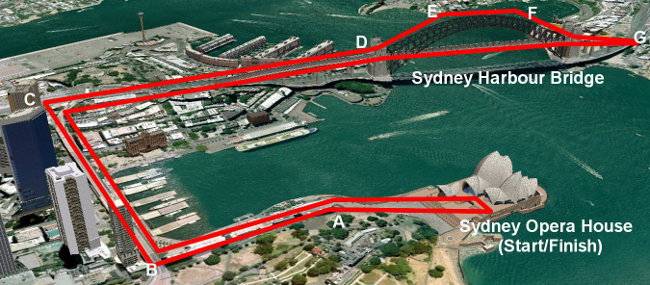# Perimeter Of Rectangles

"Peri" means "around", and "meter" means "measure".

When calculating the length of a fence around a farm, the length of a frame around a picture, or the length of the cornice around the ceiling of a room, it is the perimeter that is worked out.

Really, to find the perimeter, all you do is to add up all the sides of the shape. However, for some shapes, there are rules such as:

• Perimeter of Rectangle = (L + W) × 2
• Perimeter of Square = S × 4

Often, we must convert length units. For example, carpenters measure in millimetres for accuracy and then, these measurements are often converted to metres when calculating costs of materials such as timber.

 LENGTH RULES CONVERTING LENGTH UNITS 10 millimetres = 1 centimetre 1000 millimetres = 1 metre 100 centimetres = 1 metre 1000 metres = 1 kilometre When converting from large unit to a small unit, you multiply. When converting from a small unit to a large unit, you divide.

## Example One - Free-Running

Free-running (previously called "par cours") is a street sport where athletic participants find the shortest course over and around obstacles in urban streetscapes. Calculate the total length around the course below.(Remember to convert all measurements to the same unit such as metres.)

• Start to A - 320 metres
• A to B - 350 metres
• B to C - 1.1 kilometres
• C to D - 1.3 kilometres
• D to E to F to G (over bridge via arch) - 1430 metres
• D to G (over bridge via road) - 1100 metres

Answer:
Course Perimeter
= 320 + 350 + 1100 + 1300 + 1430 + 1100 + 1300 + 1100 + 350 + 320
= 3060 m or 3.06 km

## Example Two - Length of Fence

Find the length of the fence around a rectangular farm with a length of 2 kilometres and a width of 500 metres.

(Check that the dimensions are in the same unit. Draw a diagram. Write the rule and the unit.)

Answer:

Perimeter of Rectangle
= (L + W) × 2
= (2000 + 500) × 2
= 2500 × 2
= 5000 m or 5 km

## Example Three - Frame around a Painting

What is the length of frame required for a square painting whose side is 20cm?

Answer:

Perimeter of Square
= S × 4
= 20 × 4
= 80 cm

## Question

A rectangle has a perimeter of 336 cm and a width of 68 metres. How long is it?

Answer
100 m

## Did You Know That...?

When you touch an electric fence and receive a shock, it is because you have completed an electric circuit with the ground. If you wear shoes with insulated rubber soles, the circuit with the ground will not be completed and you will not be shocked.

Warning! Don't try this! The fence could be high voltage or you may have incorrect footwear.